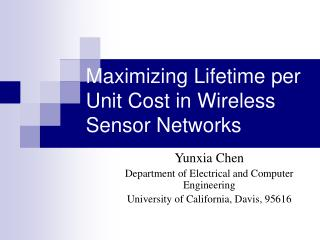# Maximizing Lifetime per Unit Cost in Wireless Sensor Networks - PowerPoint PPT PresentationDownload PresentationMaximizing Lifetime per Unit Cost in Wireless Sensor Networks

Maximizing Lifetime per Unit Cost in Wireless Sensor NetworksDownload Presentation## Maximizing Lifetime per Unit Cost in Wireless Sensor Networks

- - - - - - - - - - - - - - - - - - - - - - - - - - - E N D - - - - - - - - - - - - - - - - - - - - - - - - - - -
##### Presentation Transcript

1. Maximizing Lifetime per Unit Cost in Wireless Sensor Networks Yunxia Chen Department of Electrical and Computer Engineering University of California, Davis, 95616

2. Outline • Wireless sensor network. • Network model. • Lifetime per unit cost. • Number of sensors and sensor placement. • Numerical results. • Conclusion. EEC 273 Computer Networks

3. Wireless Sensor Networks • Sensors: low-cost, low-power, energy-constrained, limited computation and communication capability. • Gateways: powerful. • Applications: • Transportation monitoring • Temperature monitoring • …… Gateway nodes Sensor nodes EEC 273 Computer Networks

4. Basic Operation • Sensors: • Monitor certain phenomenon. • Report to the gateway nodes. • Event-driven: triggered by the event of interest. • Demand-driven: triggered by the request from the gateway nodes. • Gateways: • Collect and process the data from sensors. • Ensure end-user can access the data. EEC 273 Computer Networks

5. Sensor Deployment • Random deployment • Battlefield or disaster areas. • Generally, more sensors are used to ensure the performance. • Deterministic deployment • Friendly or accessible environment. • Optimal sensor deployment schemes which maximize the lifetime of the network or the coverage of the network. EEC 273 Computer Networks

6. Network Model • An event-driven linear wireless sensor network. • Each sensor monitors the region between itself and its right neighbor. • Generates and sends a packet to its left neighbor when an event occurs. • Packets are replayed one after another to the gateway. • The event of interest is a Poisson random process. EEC 273 Computer Networks

7. Two Questions • How many sensors should we use? • How should we place these sensors? EEC 273 Computer Networks

8. Definitions • : maximum coverage area of the network. • : maximum sensing region of each sensor. • : mean arrival rate of the event. • : distance to the gateway node . • : initial energy of each sensor. • : energy required to transmit one packet over 1m. • The energy required to transmit one packet over m distance is where is the path loss exponent. • : energy required to keep sensors alive. EEC 273 Computer Networks

9. Network Lifetime • Sensor lifetime: the amount of time until the sensor runs out of energy. • Network lifetime: the amount of time until the first sensor in the network runs out of energy. • Given the number of sensors, what is the maximum network lifetime? EEC 273 Computer Networks

10. Motivation • Different schemes are developed to maximize the network lifetime with N sensors. • Network lifetime can be increased by dividing the sensors into several small groups and enabling one group each time. • 4N -> 4T • 2N + 2N -> 6T • How many sensors should we enable each time? EEC 273 Computer Networks

11. Lifetime per Unit Cost • Definition: network lifetime divided by the number of sensors. • Characterizes the rate at which the network lifetime increases as the number of sensors increases. • Optimal number of sensors in each group = the number of sensors that maximizes the lifetime per unit cost. EEC 273 Computer Networks

12. Greedy Deployment Scheme • Intuitively, the network lifetime is maximized when all the sensors run out of energy at the same time. • Greedy sensor placement scheme depends on the number of sensors. • Maximizing network lifetime = Minimizing the transmission energy [Cheng et. al. 2004]. • Maximizing lifetime per unit cost = Minimizing total energy consumption. . EEC 273 Computer Networks

13. Average Energy Consumption • The average energy consumption of each sensor per unit time depends on the sensor placement of the network. EEC 273 Computer Networks

14. Problem Formulation • Given the coverage area , what is the number of sensors and the corresponding deployment scheme that maximizes the lifetime per unit cost? • A multivariate non-linear optimization problem: EEC 273 Computer Networks

15. Numerical Results • unit per packet over distance 1 m. • All the energy quantities are normalized by . • units. • . • . • . EEC 273 Computer Networks

16. Sensor Placement EEC 273 Computer Networks

17. Lifetime per Unit Cost EEC 273 Computer Networks

18. Optimal Number of Sensors EEC 273 Computer Networks

19. Conclusion • We observed that network lifetime can be increased by dividing sensors into small groups and enabling one group each time. • We proposed a new performance metric, the lifetime per unit cost. • We studied the number of sensors and the sensor deployment scheme that maximizes the lifetime per unit cost. • Enable small number of sensors when the mean arrival rate of the event is low or the sensing energy consumption is small. EEC 273 Computer Networks

20. Thanks! EEC 273 Computer Networks